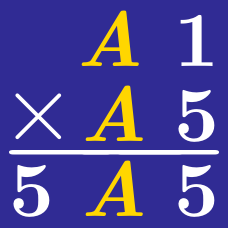Logic

# Cryptograms: Level 3 Challenges

$\large{\begin{array}{ccccccc} && & & \color{#3D99F6}X& \color{#3D99F6}X & \color{#3D99F6}X&\color{#3D99F6}X\\ && & & \color{#20A900}Y& \color{#20A900}Y & \color{#20A900}Y&\color{#20A900}Y\\ +&& & & \color{#D61F06}Z& \color{#D61F06}Z & \color{#D61F06}Z&\color{#D61F06}Z\\ \hline & & & \color{#20A900}Y& \color{#3D99F6}X& \color{#3D99F6}X & \color{#3D99F6}X&\color{#D61F06}Z\\ \hline \end{array}}$

If $\color{#3D99F6}X$, $\color{#20A900}Y$ and $\color{#D61F06}Z$ are distinct digits in the sum above, then find $\color{#D61F06}Z$.

$\begin{array} { l l l l l l } & & A & B & C & D & E \\ \times & & & & & & 4 \\ \hline & & E & D & C & B & A \\ \end{array}$

Once there was a pharaoh who had a dream. He wanted someone to interpret it. All failed to do it. Could you interpret it correctly?

The pharaoh, in his dream, saw the above multiplication.

Could you figure it out and tell us what the five digit number $\overline{ABCDE}$ is?

$\large{\begin{array}{ccccc} & F & O& R & T&Y\\ &&& T& E&N\\ +&&&T&E&N\\ \hline & S& I& X & T&Y \ \end{array}}$ Solve the above cryptarithm given than each letter represent distinct single non-negative integers.

Enter your answer as $\overline{FORTY} + \overline{TEN} + \overline{SIXTY}$.

$\frac { \begin{matrix} & A & B & C & D & E \\ \times & & & & 1 & 2 \end{matrix} }{ C\quad D\quad E\quad 0\quad A\quad B }$

Find $\overline { ABCDE }$.

$\large{\begin{array}{ccccccc} && 7& 4& 2& 5 & 8&6\\ +&&8 & 2 & 9& 4 & 3&0\\ \hline & 1& 2& 1 & 2& 0 & 1&6\\ \hline \end{array}}$

Obviously, the above addition is incorrect.

However, the display can be corrected simply by changing one digit $d$, wherever it occurs, to another digit $e$. Find the value of $d+e$.

×